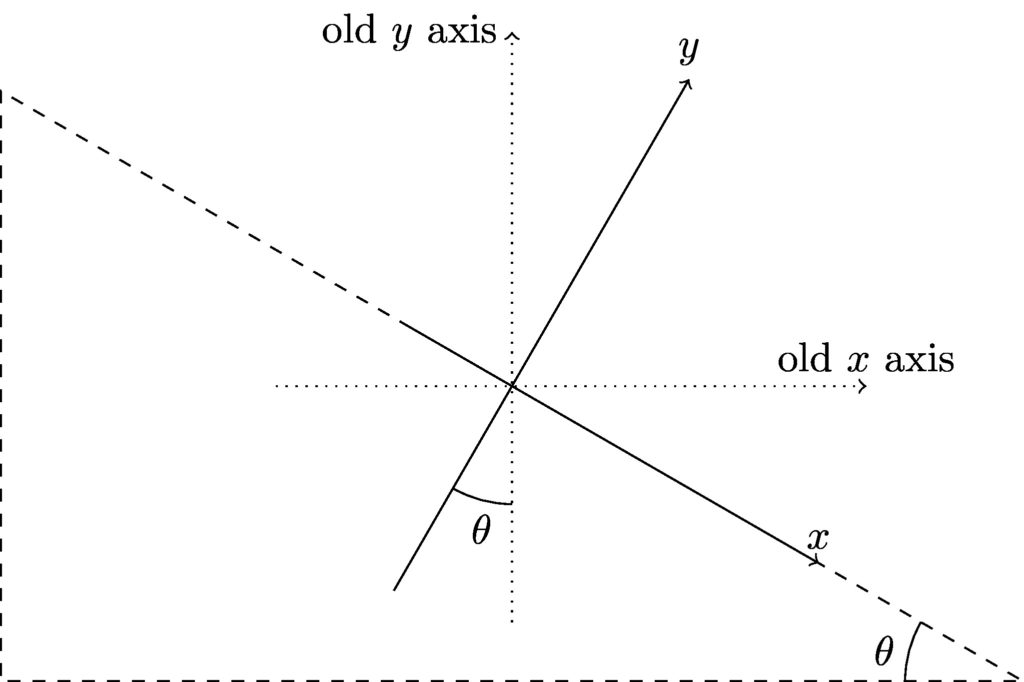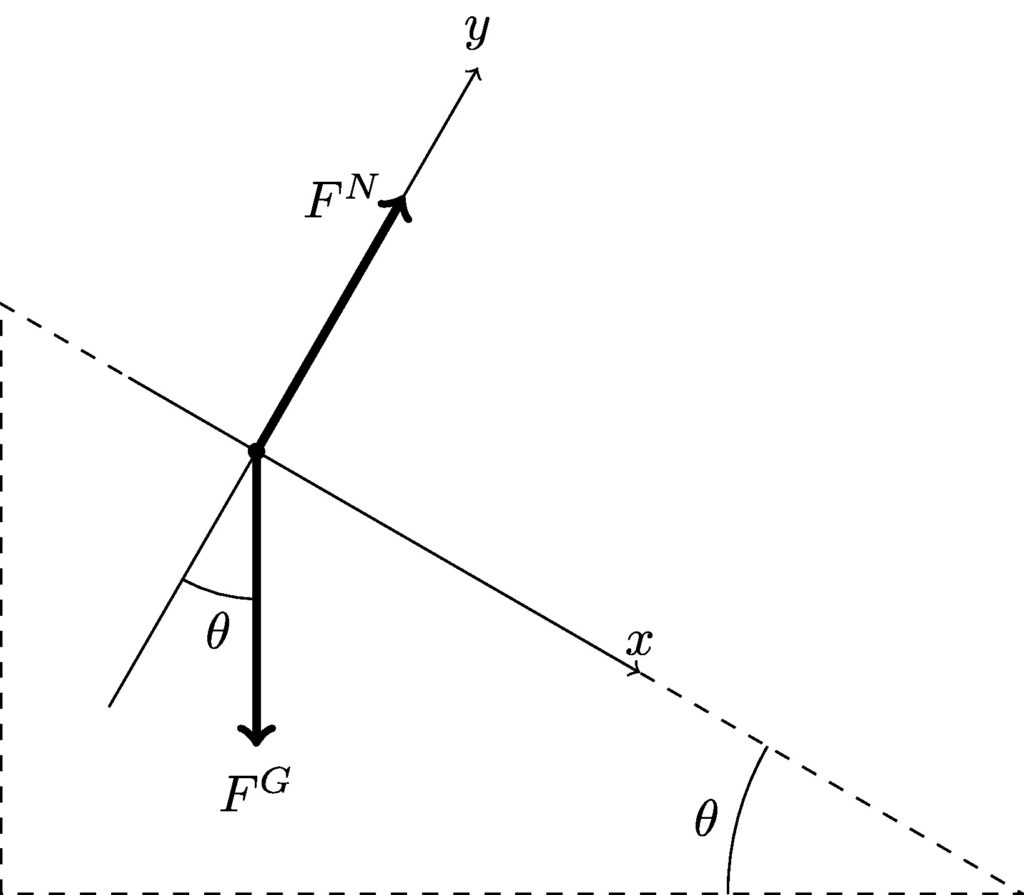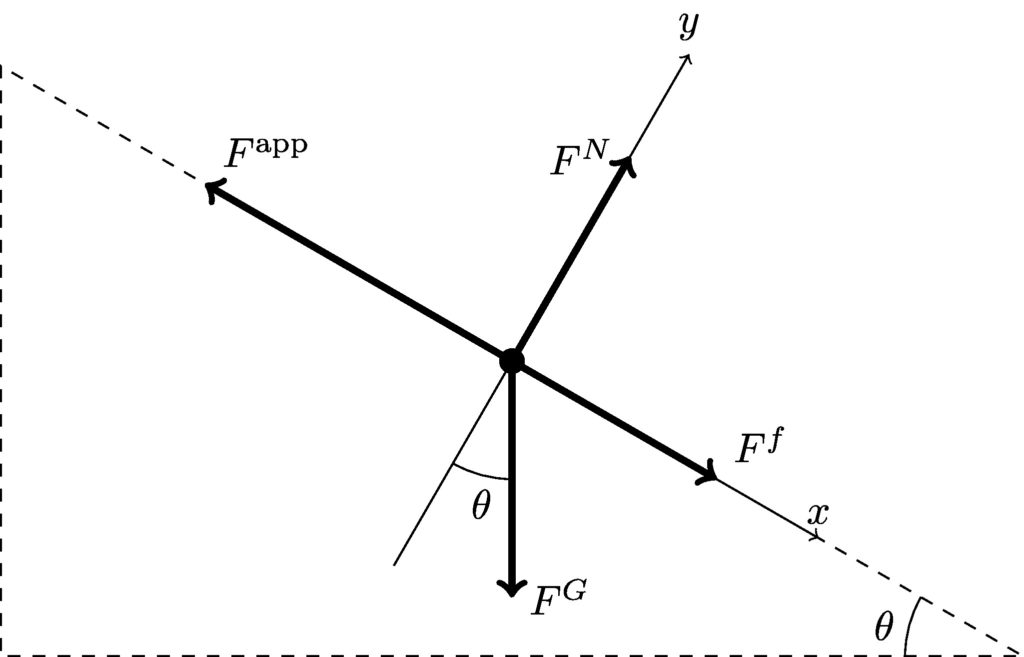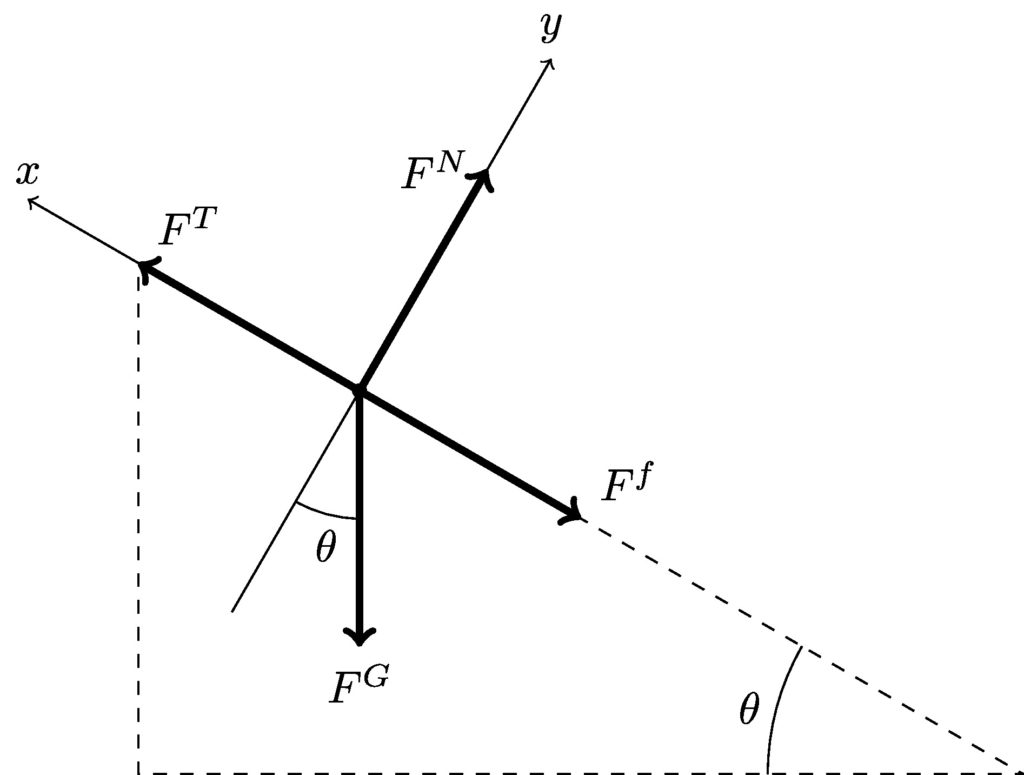# Chapter 9: Force

## 9.6 Inclined planes

Let’s say you have a box of snakes sliding down a frictionless inclined plane, and you want to know the acceleration. Of course, you begin by drawing your free body diagram:

Then you apply Newton’s second law:

\begin{align*} F_\textit{net,x} &= ma_x & F_\textit{net,y} &= ma_y \\ F^N\sin\theta &= ma_x & F^N\cos\theta – mg &= ma_y \end{align*}

Even if we know the mass of the box and the angle of the incline, we still don’t have enough information to solve this! We do not know the magnitude of the normal force of the incline pushing on the box, nor do we know either component of the acceleration. There are three unknowns, but only two equations—this is not a solvable system.

You are free to define your coordinate system however you like. For inclined plane problems, rotate your coordinate axes to simplify the problem: orient the coordinates so that the $$x$$ axis lies along the incline, as shown below. That way, any acceleration is only along the $$x$$ axis and the normal force will lie along the $$y$$ axis. You will need to use trig to find $$x$$ and $$y$$ components of the force of gravity, which still points directly downward—you can choose whatever coordinate grid you like, but you cannot change the direction of gravity!Rotated axes for an inclined plane. Consider complementary angles to determine the relationship between the angle $$\theta$$ and the new (rotated) axes.

Now let’s return to the snakes, and find out their acceleration down the plane.

#### Example 9.7

You set a box of snakes at the top of a plane that is inclined at 30°. What is the box’s acceleration?

I’ll lay the coordinate axes directly on the FBD so that we can really see the relationship between the forces acting on the box and the angle of the incline. Also, I’ll define the $$+x$$ direction as pointing down the ramp; this is just a matter of convenience so that the acceleration comes out positive.

##### FBD—Box of snakesFree body diagram for a box of snakes on an inclined plane with minimal friction; the coordinate axes are rotated.
##### Newton’s second law

\begin{align*} F_\textit{net,x} &= ma_x \\ F^G_x &= ma_x \\ F^G\sin\theta &= ma_x \\ mg\sin\theta &= ma_x \\ a_x &= g\sin\theta \\ &= \left(9.81\ \textrm{m/s}^2\right)\sin(30^\circ) \\ &= 4.9\ \textrm{m/s}^2 \end{align*}

As an aside, notice that the acceleration of the box only depends on the angle of the incline—it is independent from the mass of the box! The 17th century scientist Galileo actually did experiments on objects rolling down inclined planes and extrapolated his results to make important discoveries about objects in free fall. We’ll revisit free fall in chapter 12.

#### Example 9.8

A force with a magnitude of 95 N applied parallel to the surface of a 20° ramp will push a 12 kg mailbag up the ramp at constant speed. What is the magnitude of the kinetic friction between the bag and the ramp? What force parallel to the ramp will push it down the ramp at a constant speed?

From the information given, we can determine the magnitude of the force of friction that acts between the mailbag and the ramp. With that knowledge, we can find the force required to push the bag down the ramp.

##### FBD—MailbagFree body diagram for a mailbag pushed up an inclined plane. Rotated coordinate axes are shown.
##### Newton’s second law

Because the bag is traveling at a constant speed, its acceleration is zero. The applied force is in the negative $$x$$ direction and the friction—opposing the motion of the mailbag—points in the positive $$x$$ direction. We also need to consider the $$x$$ component of the weight of the bag.

\begin{align*} F_\textit{net,x} &= ma_x \\ F^f – F^\textit{app} + F^G_x &= m(0) \\ F^f – F^\textit{app} + F^G\sin\theta &= 0 \\ F^f &= F^\textit{app} – mg\sin\theta \\ &= (95\ \textrm{N}) – (12\ \textrm{kg})\left(9.81\ \textrm{m/s}^2\right)\sin(20^\circ) \\ &= 54.7\ \textrm{N} \end{align*}

Now that we know the friction, let’s look at the forces when the bag is being pushed down the ramp. The free body diagram will look very similar, but with the applied force and the force of friction pointing in opposite directions as before.

\begin{align*} F_\textit{net,x} &= ma_x \\ F^\textit{app} – F^f + F^G_x &= m(0) \\ F^\textit{app} – F^f + F^G\sin\theta &= 0 \\ F^\textit{app} &= F^f – mg\sin\theta \\ &= (54.7\ \textrm{N}) – (12\ \textrm{kg})\left(9.81\ \textrm{m/s}^2\right)\sin(20^\circ) \\ &= 14.4\ \textrm{N} \end{align*}

#### example 9.9

A 205 kg log is pulled up a ramp by means of a rope that is parallel to the surface of the ramp. The ramp is inclined at 30° with respect to the horizontal. The kinetic frictional force is 1700 N, and the log has an acceleration of 0.8 m/s2. If your rope is rated to 2000 N, will you be able to pull the log with this rope?

##### FBD—LogFree body diagram for a log being pulled up an inclined plane. Rotated coordinate axes are shown.

Note that I have defined $$+x$$ to point up the ramp this time; it is generally most convenient to define $$+x$$ in the direction of motion.

##### Newton’s second law

\begin{align*} F_\textit{net,x} &= ma_x \\ F^T – F^f – F^G_x &= ma_x \\ F^T – F^f – F^G\sin\theta &= ma_x \\ F^T &= ma_x + F^f + mg\sin\theta \\ &= (205\ \textrm{kg})\left(0.8\ \textrm{m/s}^2\right) + (1700\ \textrm{N}) + (205\ \textrm{kg})\left(9.81\ \textrm{m/s}^2\right)\sin(30^\circ) \\ &= 2.87\ \textrm{kN} \end{align*}

The tension in the rope would need to be greater than 2 kN, so this rope would break. You need a stronger rope.